# Inter Maths solutions for Trigonometric Ratios upto Transformations Exercise 6(c)

Intermediate first year mathematics 1A text book exercise 6(c) solutions for all problems.

These solutions are very easy to understand. Study the text book lesson very well.

Observe the example problems and solutions in the text book. Try them.

Observe the given solutions and try them in your own method.

You can see the text book solutions for

Inter Maths 1A textbook solutions

Inter Maths 1B textbook solutions

Inter Maths IIA textbook solutions

Inter Maths IIB textbook solutions

Trigonometric Ratios upto Transformations

Exercise 6(a)

Exercise 6((b)

Exercise 6(c)

Exercise 6(d)

Exercise 6(e)

Exercise 6(f)

M

## Chapter 6 Trigonometric Ratios upto Transformations

Exercise 6(c)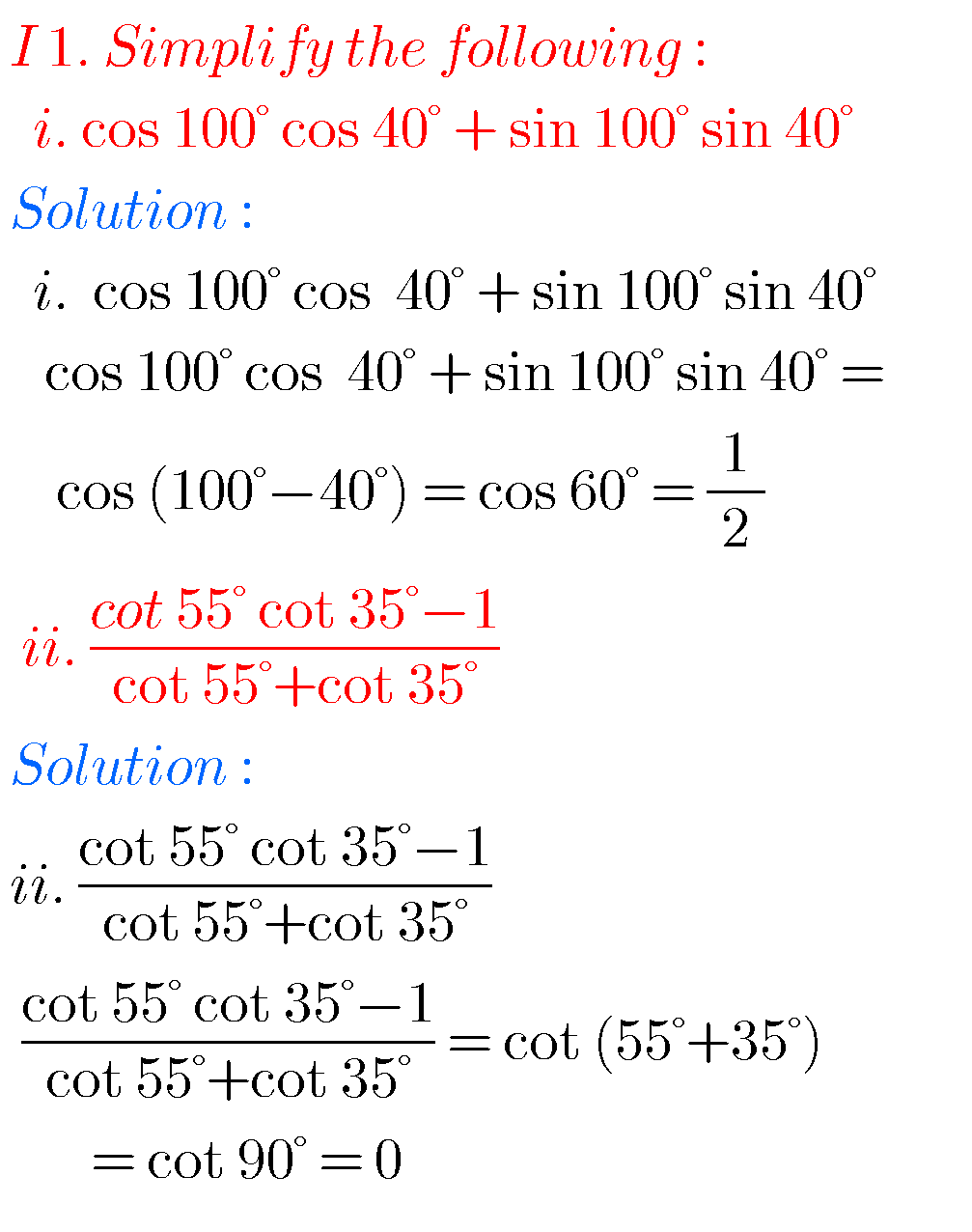##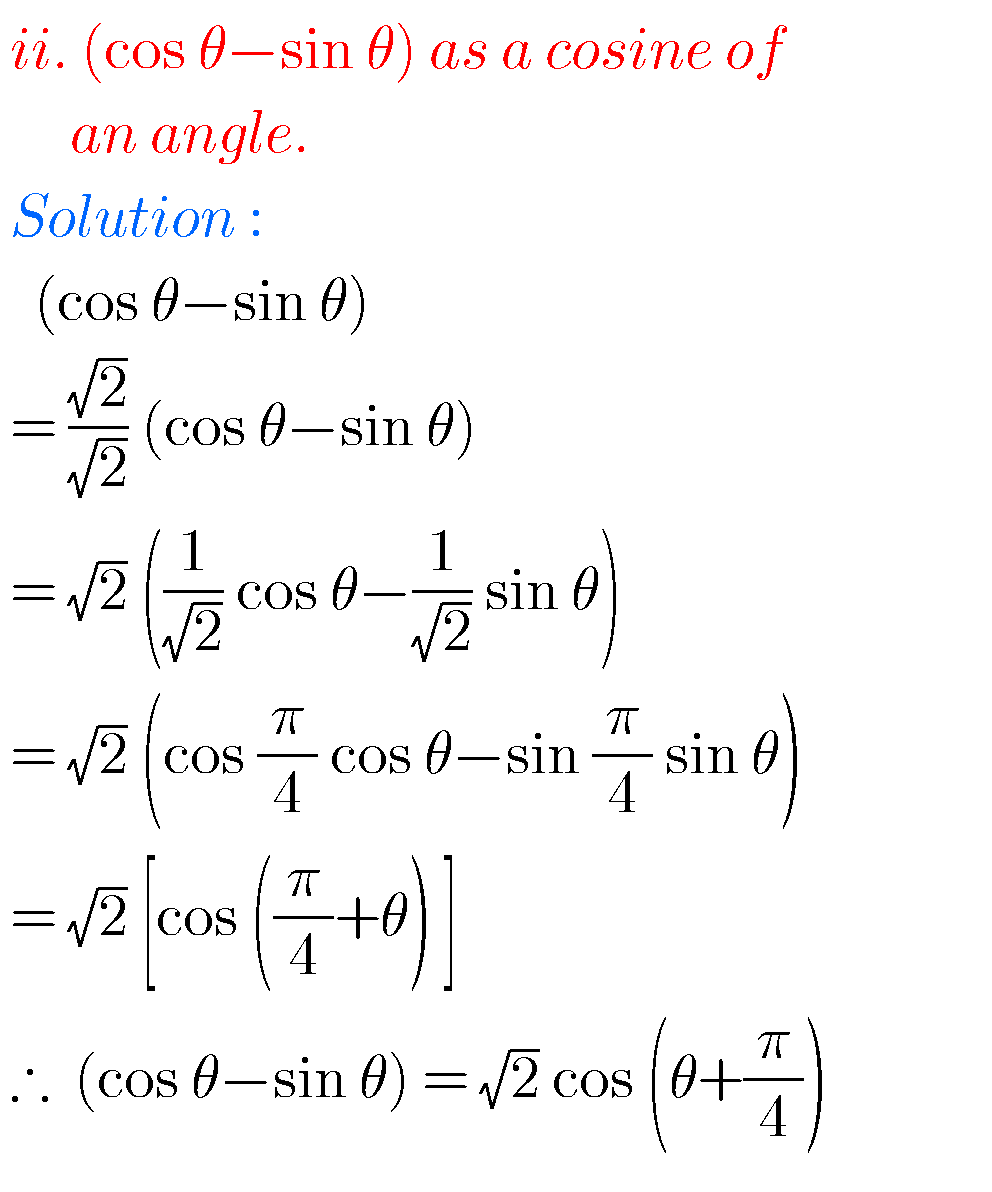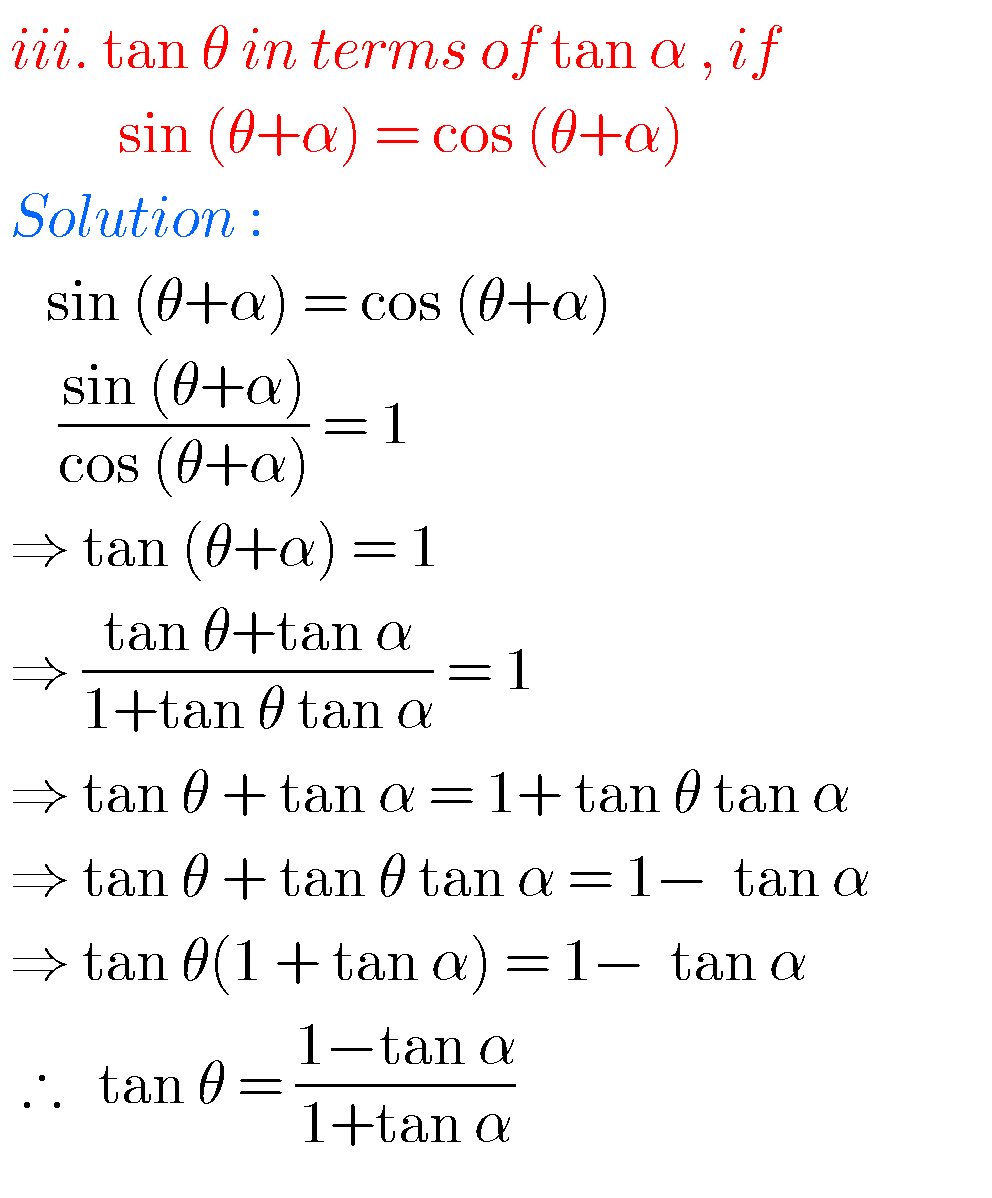##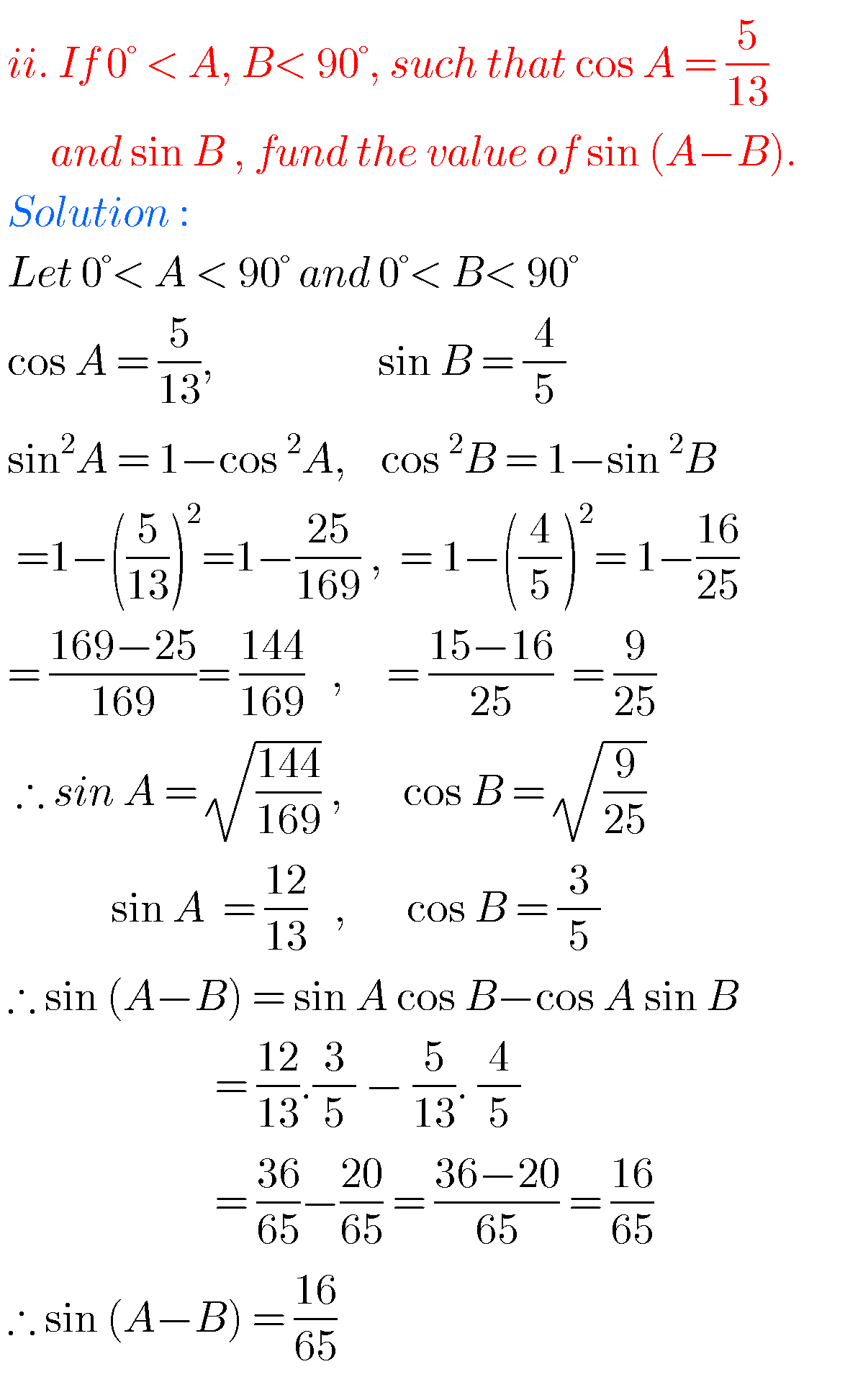##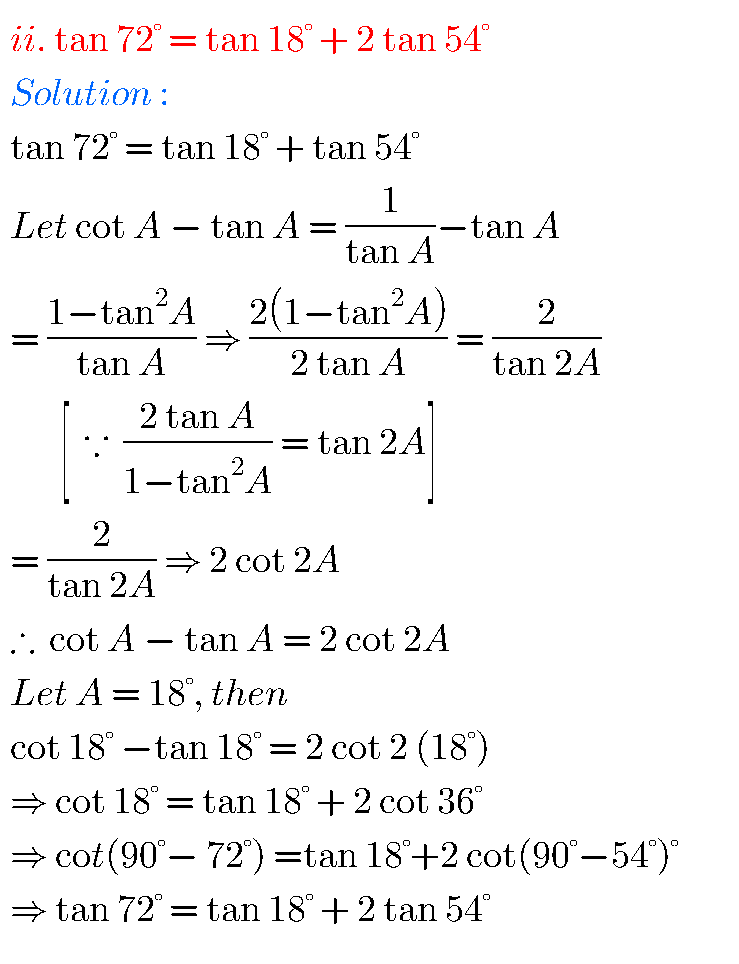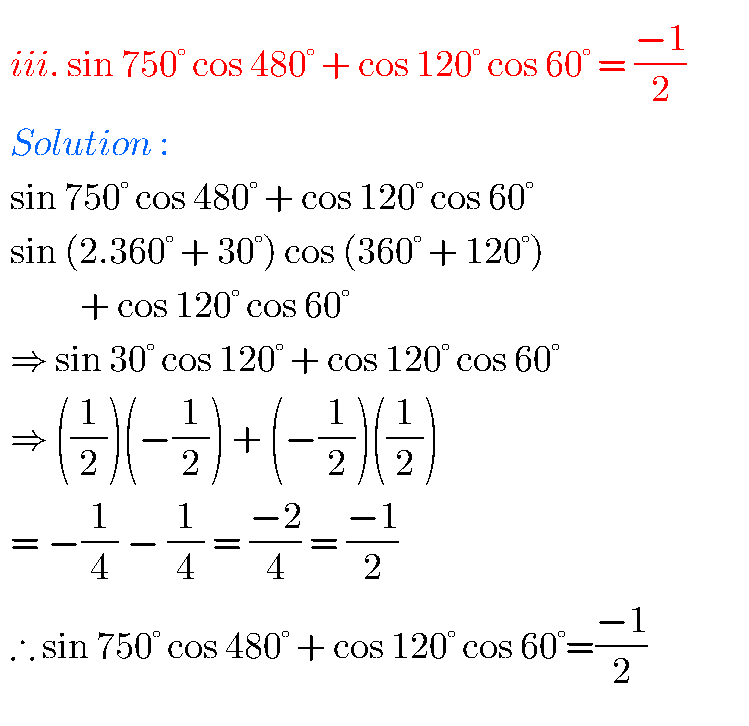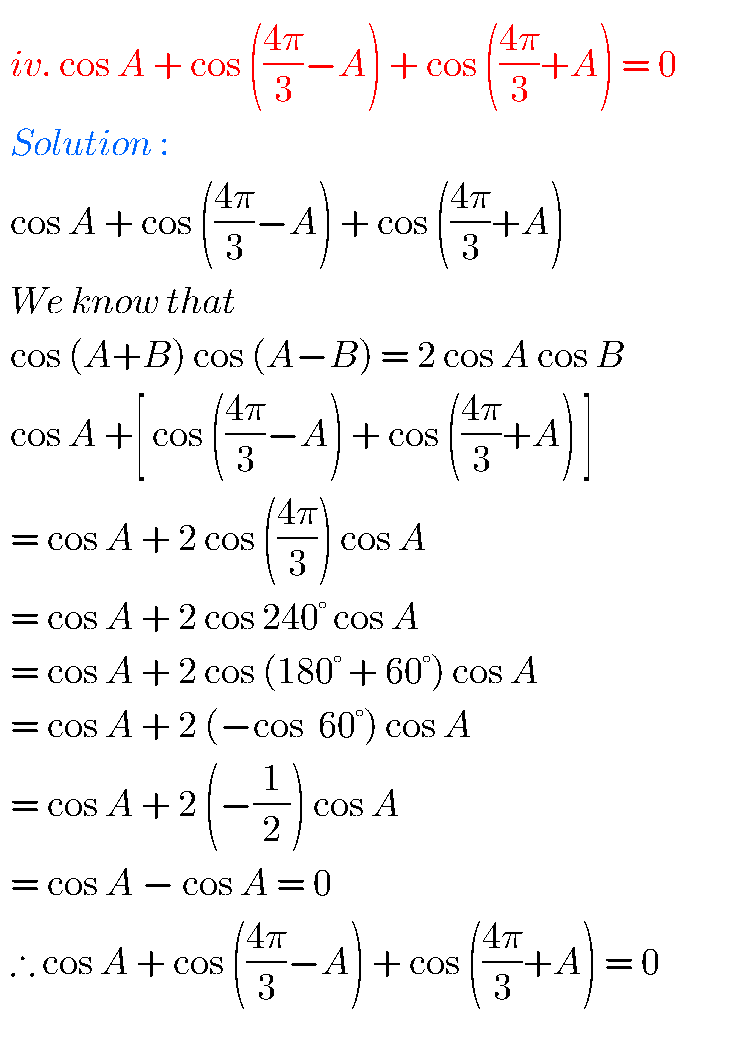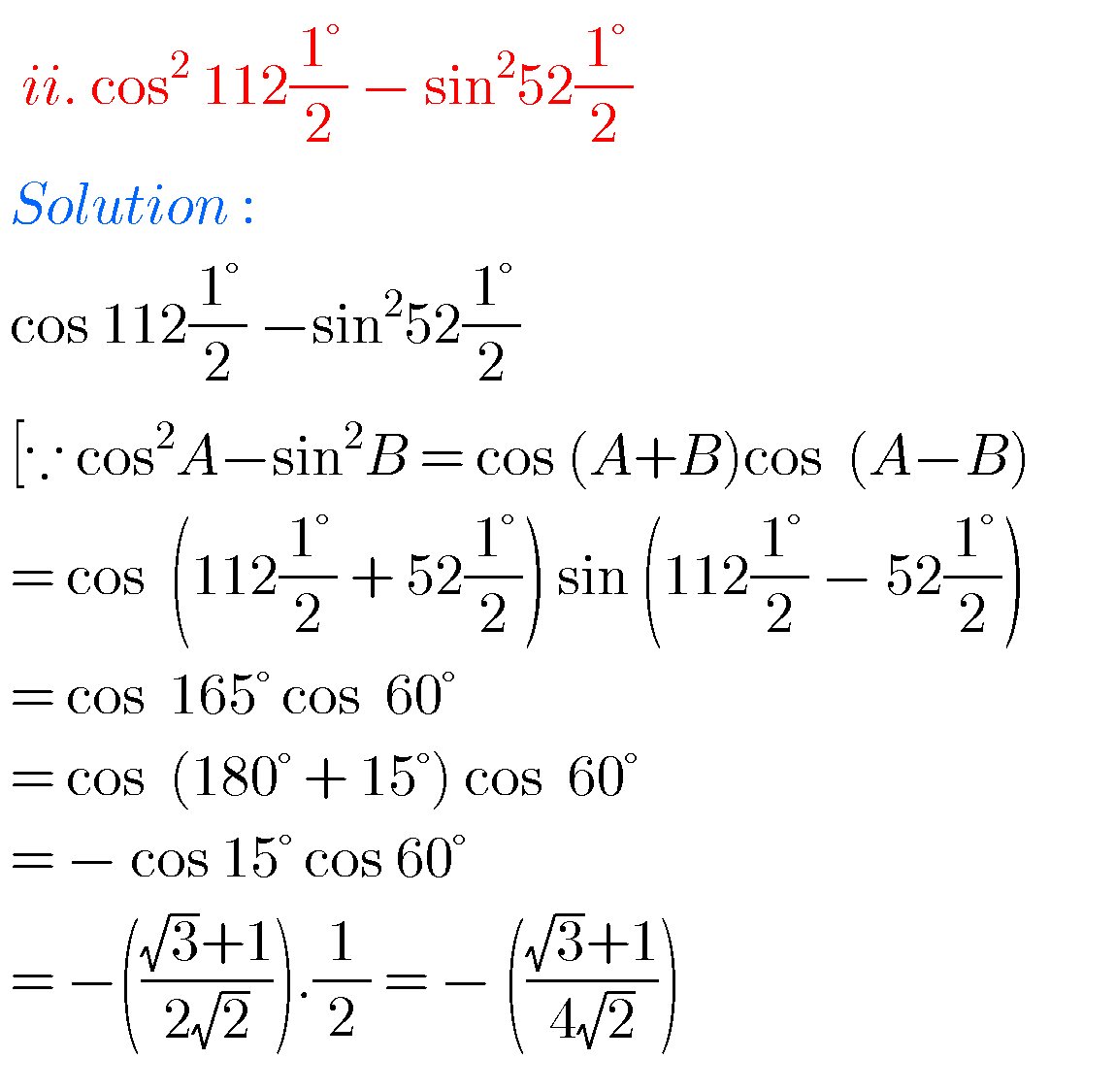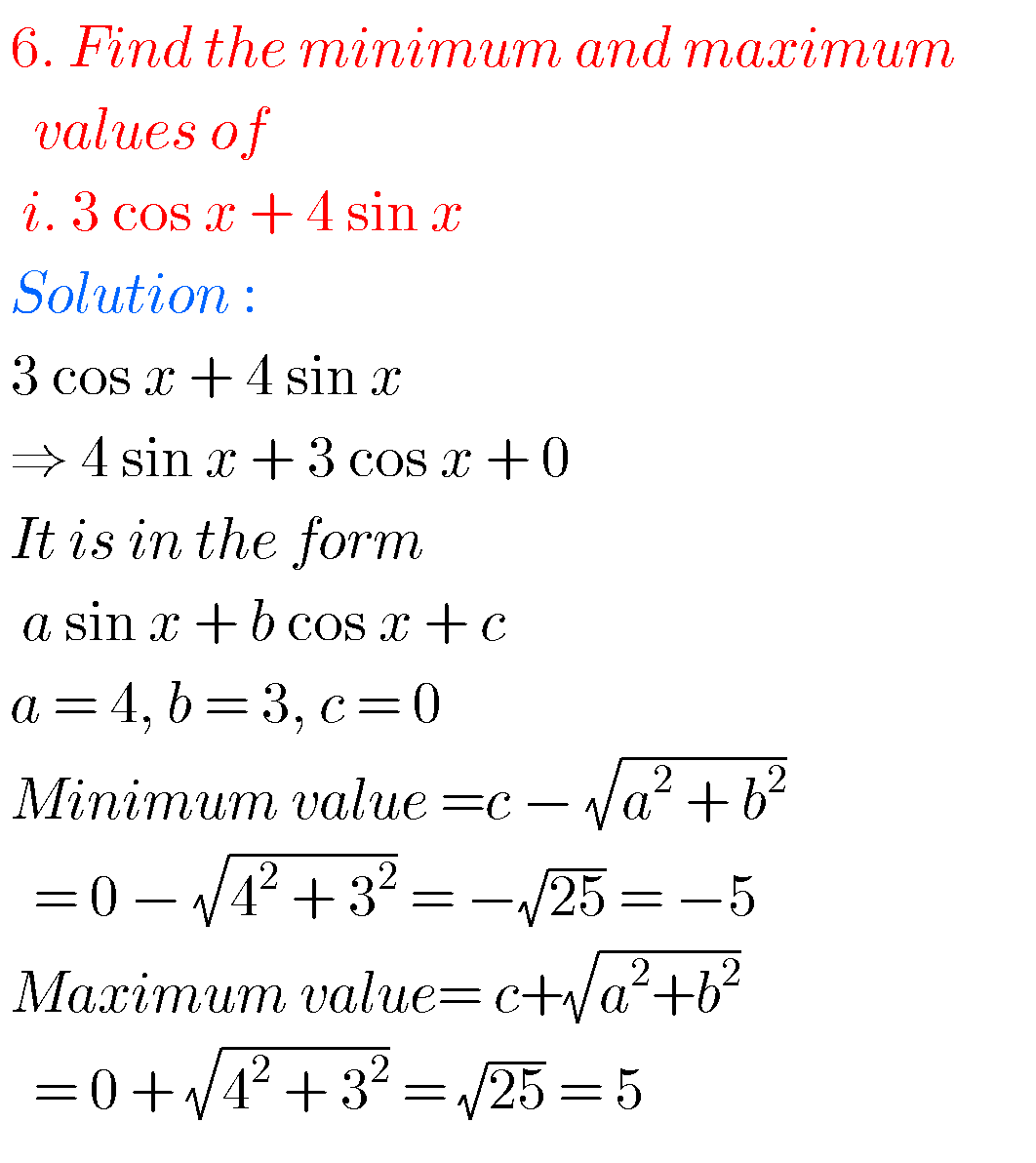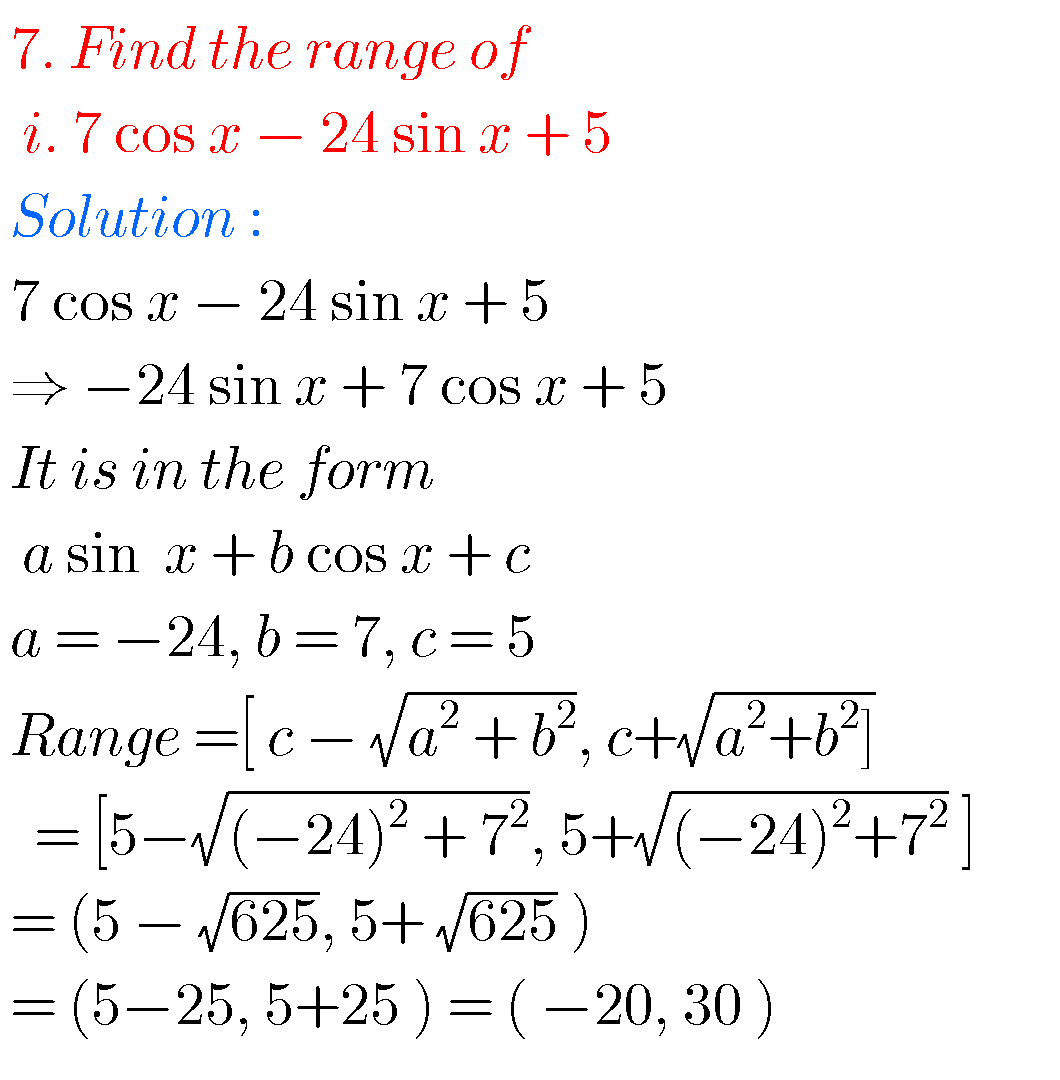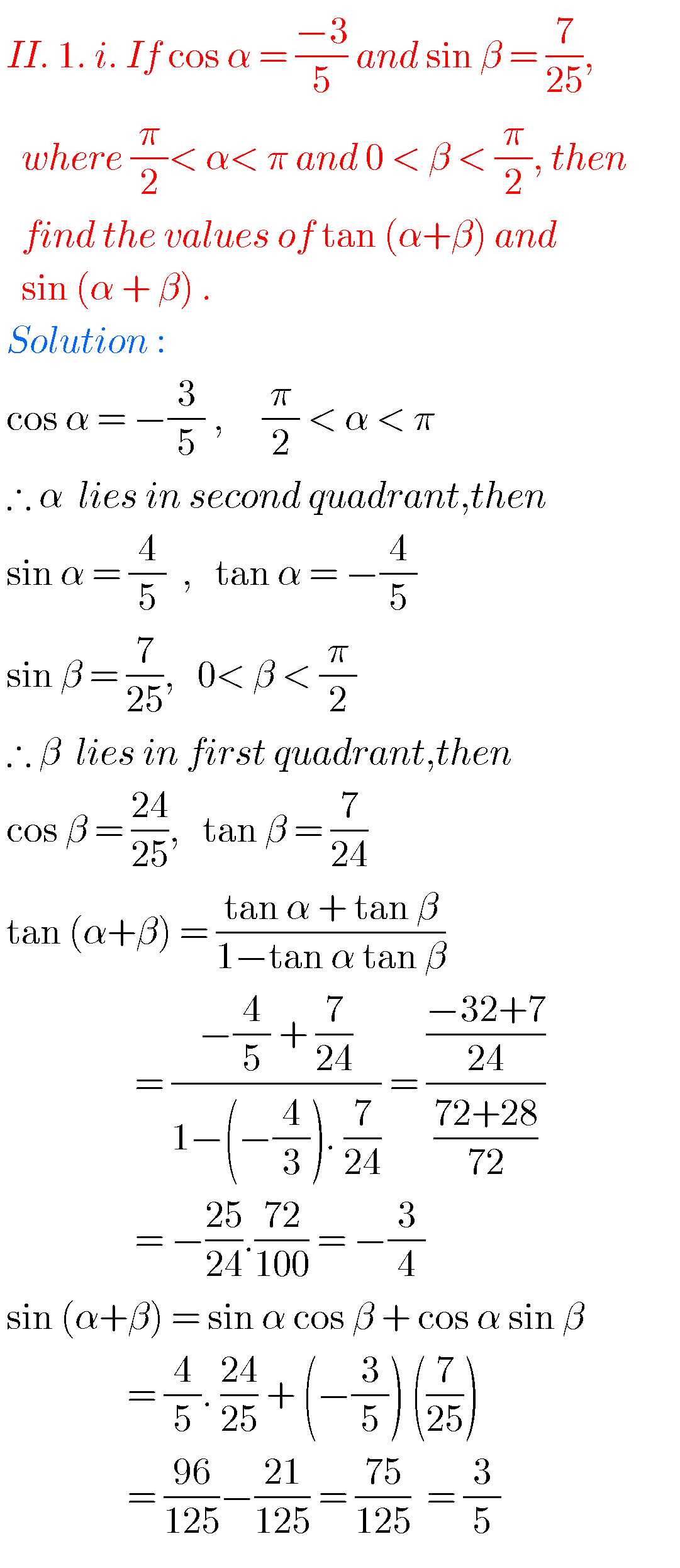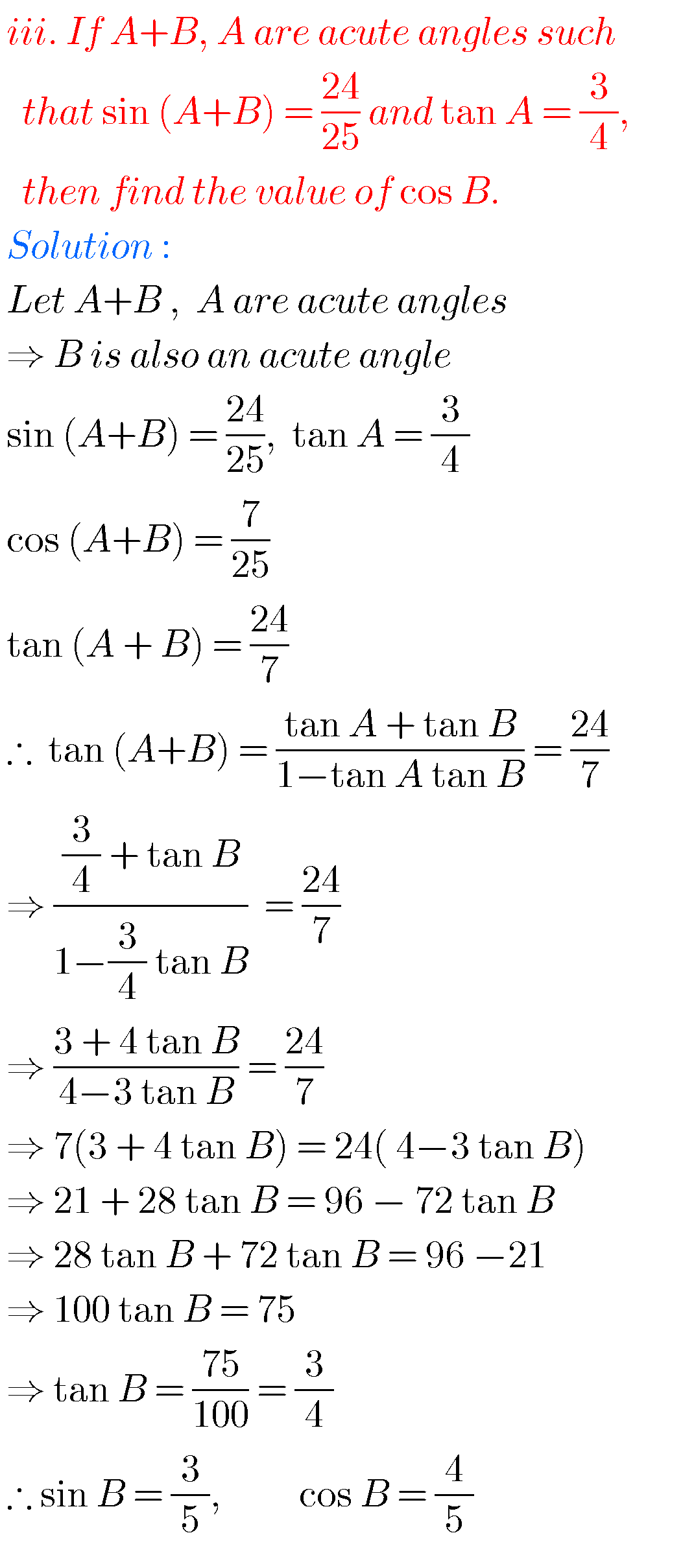## Intermediate maths 1a exercise 6(c) solutions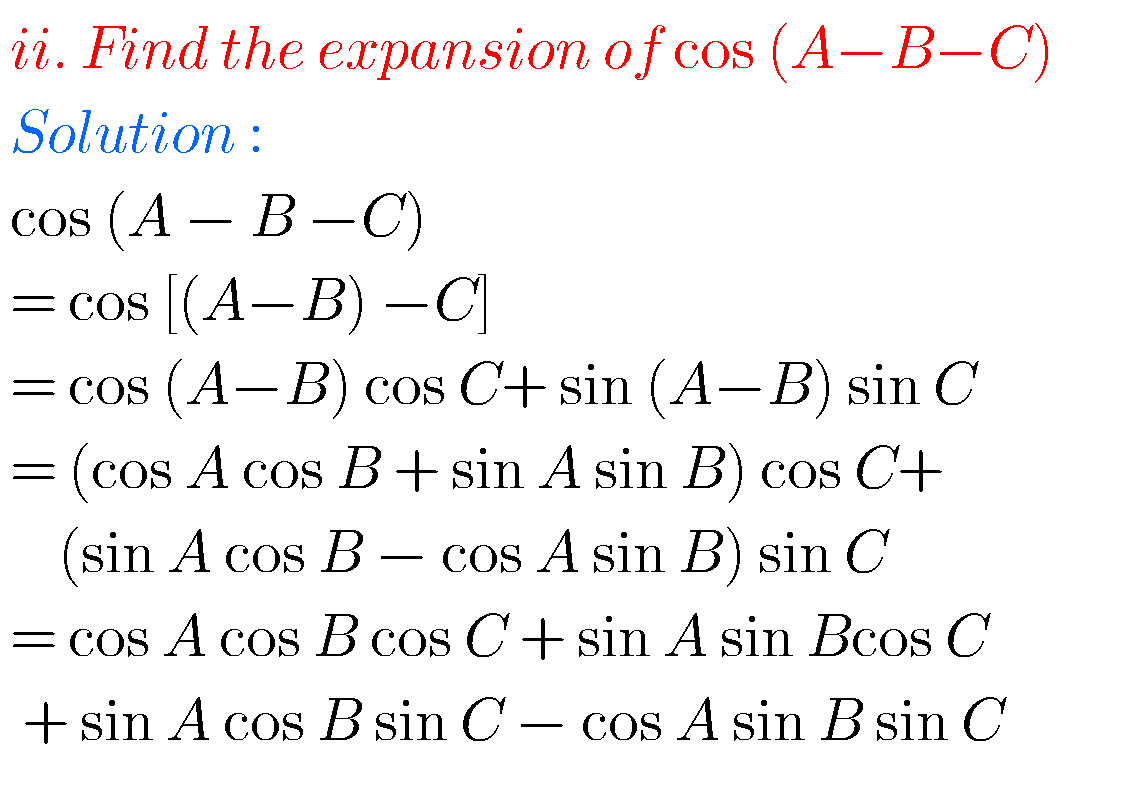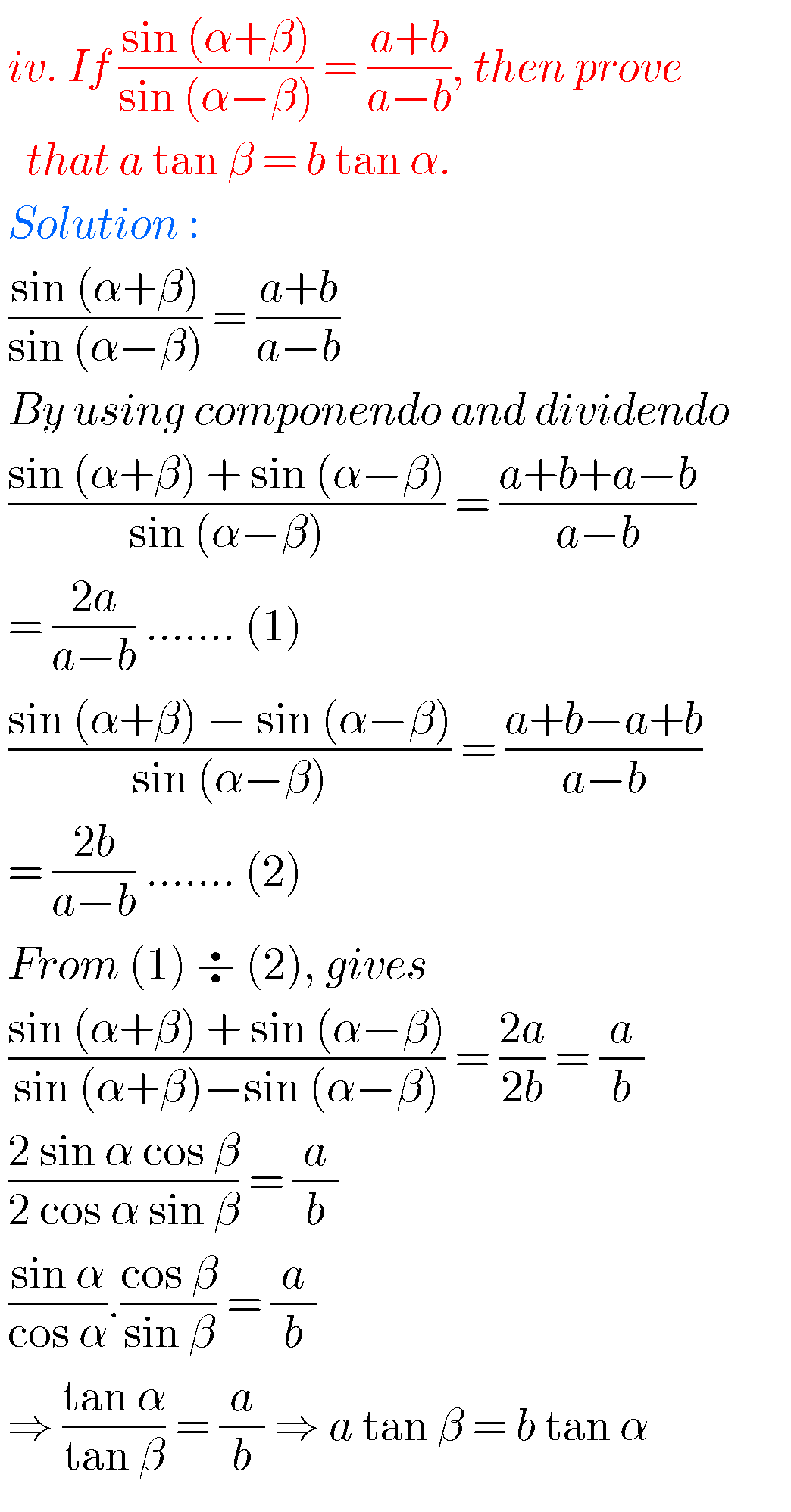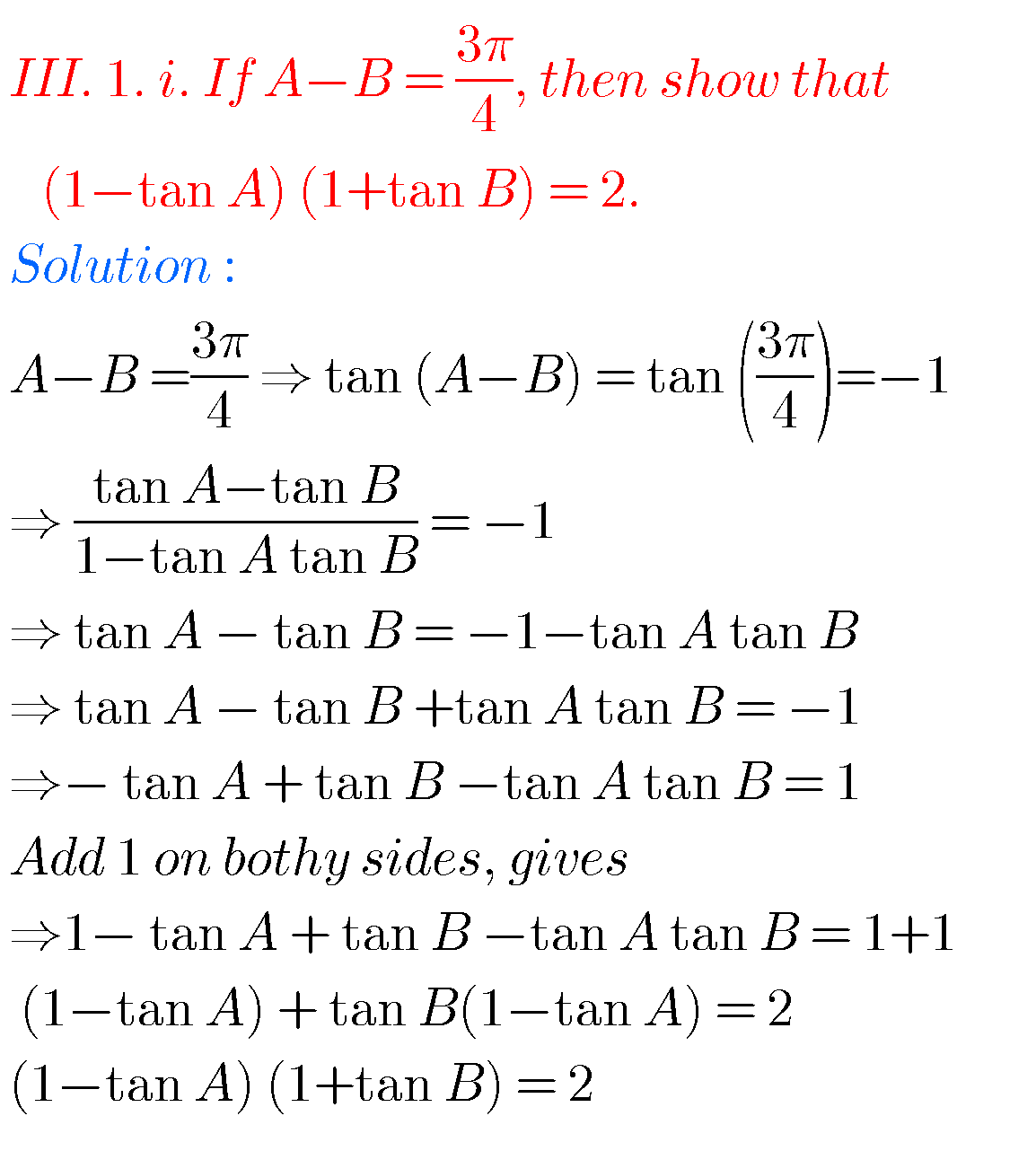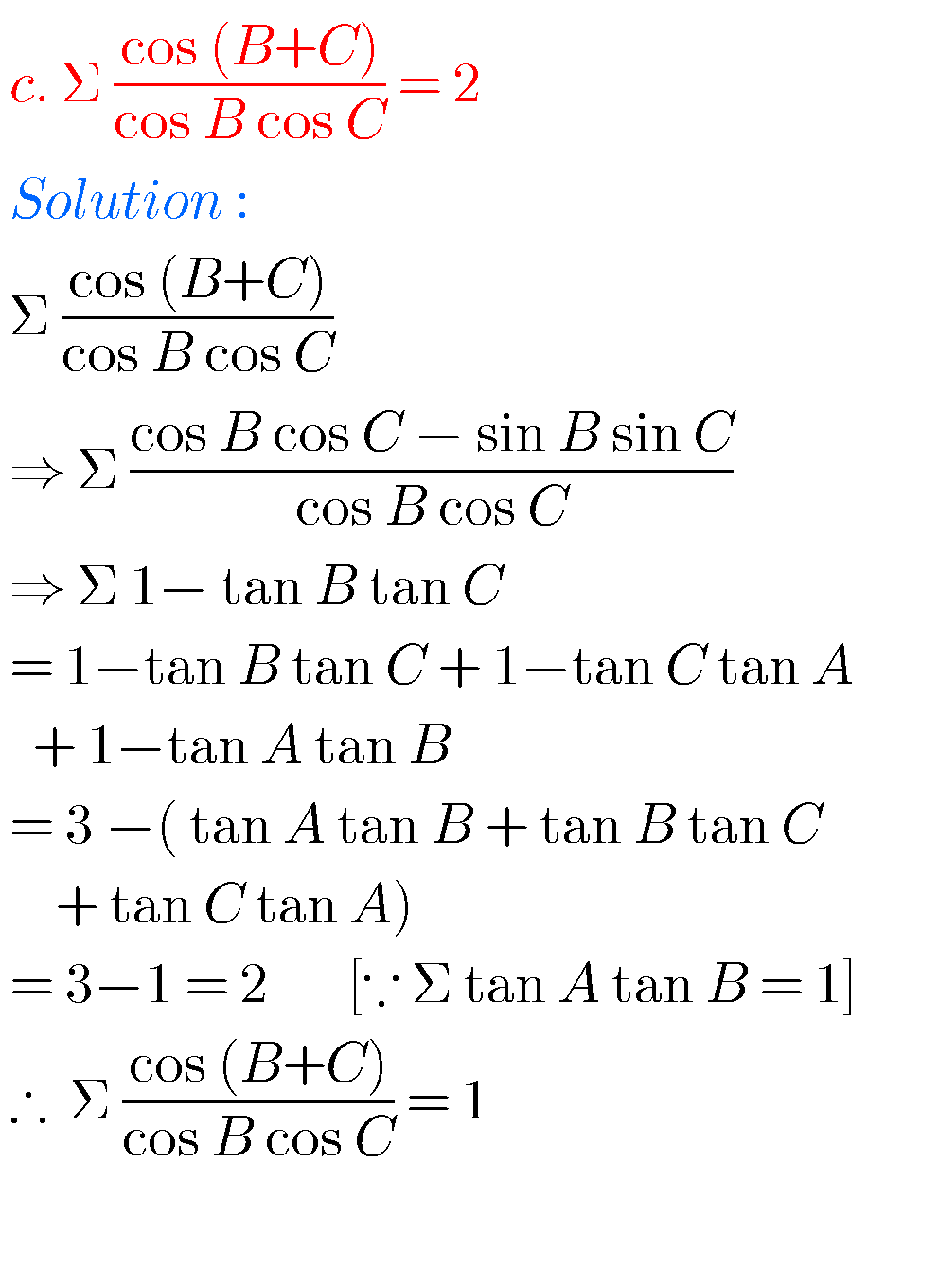Note : Observe the solutions and try them in your own methods.

Some otherYou can also see the solutions for inter maths 1A and 1B for examination purpose.

3. Matrices

You can see

Ncert maths class 6 chapter 14 solutions

Nios maths 311 book 1 Sets 1.1 solutions

### 3 thoughts on “Intermediate maths solutions for Trigonometric Ratios upto Transformations Exercise 6(c)”

1.1.1.